# Best linear method

(diff) ← Older revision | Latest revision (diff) | Newer revision → (diff)

With respect to the approximation of elements in a given set, the linear method that yields the smallest error among all linear methods. In a normed linear space, a linear method for the approximation of elements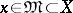by elements of a fixed subspace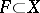is represented by a linear operator that maps the entire space, or some linear manifold containing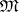, into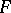. If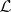is the set of all such operators, a best linear method for(if it exists) is defined by an operator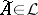for which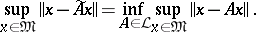The method defined by an operatorinwill certainly be a best linear method forrelative to the approximating setif, for all,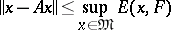(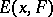is the best approximation ofby) and if, moreover, for all,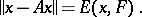The latter is certainly true ifis a Hilbert space,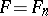is an-dimensional subspace of,andis the orthogonal projection onto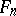, i.e.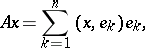where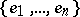is an orthonormal basis in.

Letbe a Banach space of functions defined on the entire real line, with a translation-invariant norm: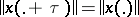(this condition holds, e.g. for the norms of the spaces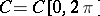and,, of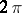-periodic functions); let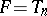be the subspace of trigonometric polynomials of order. There exist best linear methods (relative to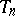) for a classof functionsthat contains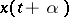for any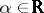whenever it contains. An example is the linear method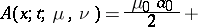(*)where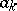and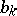are the Fourier coefficients of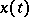relative to the trigonometric system, and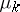and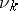are numbers.

Now consider the classes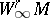(and),of-periodic functionswhose derivatives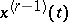are locally absolutely continuous and whose derivatives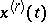are bounded in norm in(respectively, in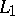) by a number. For these classes, best linear methods of the type (*) yield the same error (over the entire class) in the metric of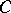(respectively,) as the best approximation by a subspace; the analogous assertion is true for these classes with any rational number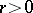(interpreting the derivativesin the sense of Weyl). For integersbest linear methods of type (*) have been constructed using only the coefficients(all).

Ifis the subspace of-periodic polynomial splines of orderand defect 1 with respect to the partition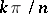,then a best linear method for the classes(and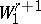),is achieved in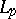,(resp. in) by splines in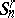interpolating the functionat the points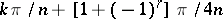.

How to Cite This Entry:
Best linear method. Encyclopedia of Mathematics. URL: http://encyclopediaofmath.org/index.php?title=Best_linear_method&oldid=19004
This article was adapted from an original article by N.P. KorneichukV.P. Motornyi (originator), which appeared in Encyclopedia of Mathematics - ISBN 1402006098. See original article